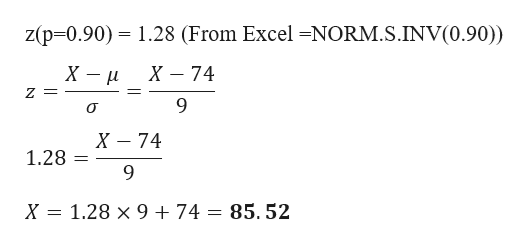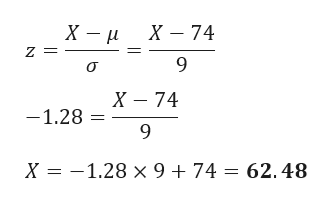# In a large section of a statistics​ class, the points for the final exam are normally​ distributed, with a mean of7474and a standard deviation of99.Grades are assigned such that the top​ 10% receive​ A's, the next​ 20% received​ B's, the middle​ 40% receive​ C's, the next​ 20% receive​ D's, and the bottom​ 10% receive​ F's. Find the lowest score on the final exam that would qualify a student for an​ A, a​ B, a​ C, and a D.

Question
1 views
In a large section of a statistics​ class, the points for the final exam are normally​ distributed, with a mean of
7474
and a standard deviation of
99.
Grades are assigned such that the top​ 10% receive​ A's, the next​ 20% received​ B's, the middle​ 40% receive​ C's, the next​ 20% receive​ D's, and the bottom​ 10% receive​ F's. Find the lowest score on the final exam that would qualify a student for an​ A, a​ B, a​ C, and a D.

check_circle

Step 1

Given data

Mean = 74

Standard Deviation = 9

a)The Lowest Score for A grade is given by

p-value will be 0.90 for top 10%

Finding the value of z at p=0.90

z(p=0.90) = 1.28 ( From Excel =NORM.S.INV(0.90))help_outlineImage Transcriptionclosez(p-0.90) 1.28 (From Excel -NORM.S.INV(0.90)) X - X 74 Z = X 74 1.28 9 X = 1.28 x 9 +74 = 85.52 fullscreen
Step 2

b)The Lowest Score for B grade is given by

p-value will be 0.70 for top 30%

Finding the value of z at p=0.70

z(p=0.70) = 0.524 ( From Excel =NORM.S.INV(0.70))

Step 3

p-value will be 0.10 for lowest 10%

Finding the value of z at ...help_outlineImage TranscriptioncloseX — и Z = X 74 9 о X 74 -1.28 9 X— — 1.28 х9+74 — 62. 48 fullscreen

### Want to see the full answer?

See Solution

#### Want to see this answer and more?

Solutions are written by subject experts who are available 24/7. Questions are typically answered within 1 hour.*

See Solution
*Response times may vary by subject and question.
Tagged in

### Other